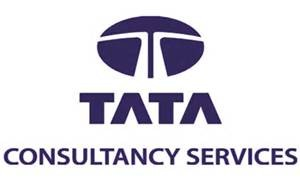1. On a 26 question test, five points were deducted for each wrong answer and eight points were added for each correct answer. If all the questions were answered, how many were correct, if the score was zero ?
a. 10
b. 12
c. 11
d. 13
Explanation:
Take options and check. If 10 are correct, his score is 10 x 8 = 80. But 16 are wrong. So total negative marking is 16 x 5 = 80. So final score is zero.
2. In how many ways a team of 11 must be selected from 5 men and 11 women such that the team must comprise of not more than 3 men?
a. 1565
b. 1243
c. 2256
d. 2456
Explanation;
The team may consist of 0 men + 11 women, 1 men + 10 women, 2 men + 9 women, or 3 men + 8 women.So Number of ways are = 11C11+5C1×11C10+5C2×11C9+5C3×11C8 = 2256

3. Given that 0 < a < b < c < d, which of the following the largest ?
a.(c+d) / (a+b)
b.(a+d) / (b+c)
c.(b+c) / (a+d)
d.(b+d) / (a+c)
Explanation: Take a = 1, b = 2, c = 3, d = 4. option A is clearly true.

4. Eesha bought 18 sharpeners for Rs.100. She paid 1 rupee more for each white sharpener than for each brown sharpener. What is the price of a white sharpener and how many white sharpener did she buy ?
a. Rs.5, 10
b. Rs.6, 10
c. Rs.5, 8
d. Rs.6, 8
Explanation: Just check the options. If she bought 10 white sharpeners at Rs.6 per piece, She has spent Rs.60 already. And with the remaining Rs.40, she bought 8 brown sharpeners at 40/8 = Rs.5 which is Rs.1 less than White sharpener.

5.The fourteen digits of a credit card are to be written in the boxes shown above. If the sum of every three consecutive digits is 18, then the value of x is :
a. 3
b. cannot be determined from the given information.
c. 2
d. 1
Explanation:
Let us assume right most two squares are a , b
Then Sum of all the squares = 18 x 4 + a + b ………. (1)
Also Sum of the squares before 7 = 18
Sum of the squares between 7, x = 18
and sum of the squares between x , 8 = 18
So Sum of the 14 squares = 18 + 7 + 18 + x + 18 + 8 + a + b…… (2)
Equating 1 and 2 we get x = 3

6. Four people each roll a four die once. Find the probability that at least two people will roll the same number ?
a. 5/18
b. 13/18
c. None of the given choices
d. 1295/1296
Explanation:
The number of ways of rolling a dice where no two numbers probability that no one rolls the same number = 6 x 5 x 4 x 3
Now total possibilities of rolling a dice = 64
The probability that a no one gets the same number = 6×5×4×364=518
So the probability that at least two people gets same number = 1?518=1318

7. Jake can dig a well in 16 days. Paul can dig the same well in 24 days. Jake, Paul and Hari together dig the well in 8 days. Hari alone can dig the well in
a. 96 days
b. 48 days
c. 32 days
d. 24 days
Explanation: Simple one. Let the total work to be done is 48 meters. Now Jake can dig 3 mts, Paul can dig 2 mts a day. Now all of them combined dug in 8 days so per day they dug 48/8 = 6 mts. So Of these 8 mts, Hari capacity is 1 mt.
So he takes 48 /1 = 48 days to complete the digging job.

8. Eesha bought 18 sharpeners for Rs.100. She paid 1 rupee more for each white sharpener than for each brown sharpener. What is the price of a white sharpener and how many white sharpener did she buy ?
a. Rs.5, 10
b. Rs.6, 10
c. Rs.5, 8
d. Rs.6, 8
Ans:
Explanation: This question can be solved easily by going through options.
A. White sharpener total cost: Rs.5 x 10 = Rs.50. Brown sharpeners cost = Rs.4 x 8 = 32. Total cost is only Rs.82. Wrong option.
B. White sharpener total cost: Rs.6 x 10 = Rs.60. Brown sharpeners cost = Rs.5 x 8 = 40. Total cost is Rs.100. Correct option.

9. The sum of the digits of a three digit number is 17, and the sum of the squares of its digits is 109. If we subtract 495 from the number, we shall get a number consisting of the same digits written in the reverse order. Find the number.
a. 773
b. 683
c. 944
d. 863
Explanation: Check options. Sum of the squares should be equal to 109. Only Options B and D satisfying. When we subtract 495, only 863 becomes 368.

10. Mark told John “If you give me half your money I will have Rs.75. John said, “if you give me one third of your money, I will have Rs.75/- How much money did John have ?
a. 45
b. 60
c. 48
d. 37.5
Explanation: Let the money with Mark and John are M and J respectively.
Now
M + J/2 = 75
M/3 + J = 75
Solving we get M = 45, and J = 60.